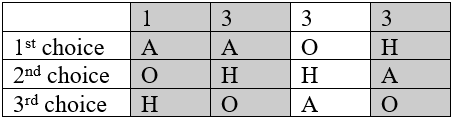$$\newcommand{\id}{\mathrm{id}}$$ $$\newcommand{\Span}{\mathrm{span}}$$ $$\newcommand{\kernel}{\mathrm{null}\,}$$ $$\newcommand{\range}{\mathrm{range}\,}$$ $$\newcommand{\RealPart}{\mathrm{Re}}$$ $$\newcommand{\ImaginaryPart}{\mathrm{Im}}$$ $$\newcommand{\Argument}{\mathrm{Arg}}$$ $$\newcommand{\norm}{\| #1 \|}$$ $$\newcommand{\inner}{\langle #1, #2 \rangle}$$ $$\newcommand{\Span}{\mathrm{span}}$$

# 2.4: What’s Wrong with Plurality?

$$\newcommand{\vecs}{\overset { \rightharpoonup} {\mathbf{#1}} }$$ $$\newcommand{\vecd}{\overset{-\!-\!\rightharpoonup}{\vphantom{a}\smash {#1}}}$$$$\newcommand{\id}{\mathrm{id}}$$ $$\newcommand{\Span}{\mathrm{span}}$$ $$\newcommand{\kernel}{\mathrm{null}\,}$$ $$\newcommand{\range}{\mathrm{range}\,}$$ $$\newcommand{\RealPart}{\mathrm{Re}}$$ $$\newcommand{\ImaginaryPart}{\mathrm{Im}}$$ $$\newcommand{\Argument}{\mathrm{Arg}}$$ $$\newcommand{\norm}{\| #1 \|}$$ $$\newcommand{\inner}{\langle #1, #2 \rangle}$$ $$\newcommand{\Span}{\mathrm{span}}$$ $$\newcommand{\id}{\mathrm{id}}$$ $$\newcommand{\Span}{\mathrm{span}}$$ $$\newcommand{\kernel}{\mathrm{null}\,}$$ $$\newcommand{\range}{\mathrm{range}\,}$$ $$\newcommand{\RealPart}{\mathrm{Re}}$$ $$\newcommand{\ImaginaryPart}{\mathrm{Im}}$$ $$\newcommand{\Argument}{\mathrm{Arg}}$$ $$\newcommand{\norm}{\| #1 \|}$$ $$\newcommand{\inner}{\langle #1, #2 \rangle}$$ $$\newcommand{\Span}{\mathrm{span}}$$

The election from Example 2 may seem totally clean, but there is a problem lurking that arises whenever there are three or more choices. Looking back at our preference table, how would our members vote if they only had two choices?

Anaheim vs Orlando: 7 out of the 10 would prefer Anaheim over OrlandoAnaheim vs Hawaii: 6 out of 10 would prefer Hawaii over AnaheimThis doesn’t seem right, does it? Anaheim just won the election, yet 6 out of 10 voters, 60% of them, would have preferred Hawaii! That hardly seems fair. Marquis de Condorcet, a French philosopher, mathematician, and political scientist wrote about how this could happen in 1785, and for him we name our first fairness criterion.

## Fairness Criteria

The fairness criteria are statements that seem like they should be true in a fair election.

## Condorcet Criterion

If there is a choice that is preferred in every one-to-one comparison with the other choices, that choice should be the winner. We call this winner the Condorcet Winner, or Condorcet Candidate.

## Example 3

In the election from Example 2, what choice is the Condorcet Winner?

Solution

We see above that Hawaii is preferred over Anaheim. Comparing Hawaii to Orlando, we can see 6 out of 10 would prefer Hawaii to Orlando.Since Hawaii is preferred in a one-to-one comparison to both other choices, Hawaii is the Condorcet Winner.

## Example 4

Consider a city council election in a district that is historically 60% Democratic voters and 40% Republican voters. Even though city council is technically a nonpartisan office, people generally know the affiliations of the candidates. In this election there are three candidates: Don and Key, both Democrats, and Elle, a Republican. A preference schedule for the votes looks as follows:

$$\begin{array}{|l|l|l|l|} \hline & 342 & 214 & 298 \\ \hline 1^{\text {st }} \text { choice } & \text { Elle } & \text { Don } & \text { Key } \\ \hline 2^{\text {nd }} \text { choice } & \text { Don } & \text { Key } & \text { Don } \\ \hline 3^{\text {rd }} \text { choice } & \text { Key } & \text { Elle } & \text { Elle } \\ \hline \end{array}$$

Solution

We can see a total of $$342 + 214 + 298 = 854$$ voters participated in this election. Computing percentage of first place votes:

Don: $$214/854 = 25.1\%$$

Key: $$298/854 = 34.9\%$$

Elle: $$342/854 = 40.0\%$$

So in this election, the Democratic voters split their vote over the two Democratic candidates, allowing the Republican candidate Elle to win under the plurality method with 40% of the vote.

Analyzing this election closer, we see that it violates the Condorcet Criterion. Analyzing the one-to-one comparisons:

Elle vs Don: 342 prefer Elle; 512 prefer Don: Don is preferred

Elle vs Key: 342 prefer Elle; 512 prefer Key: Key is preferred

Don vs Key: 556 prefer Don; 298 prefer Key: Don is preferred

So even though Don had the smallest number of first-place votes in the election, he is the Condorcet winner, being preferred in every one-to-one comparison with the other candidates.

## Try it Now 2

Consider the election from Try it Now 1. Is there a Condorcet winner in this election?

Determining the Condorcet Winner:

G vs M: $$44+14+20 = 78$$ prefer G, $$70+22+80=172$$ prefer M: M preferred

G vs B: $$44+14+20+70=148$$ prefer G, $$22+80+39 = 141$$ prefer B: G preferred

M vs B: $$44+70+22=136$$ prefer M, $$14+80+39=133$$ prefer B: M preferred

M is the Condorcet winner, based on the information we have.

2.4: What’s Wrong with Plurality? is shared under a CC BY-SA 3.0 license and was authored, remixed, and/or curated by David Lippman via source content that was edited to conform to the style and standards of the LibreTexts platform; a detailed edit history is available upon request.